##Isogonal transformation as a quadratic one

Quadratic transformations are related to families of conics. Given a family of conics, and a point P, all polars of P with respect to the conic-members of the family pass through another point P' = F(P). This defines the quadratic transformation of the family. In practice, P' is constructed as the intersection point of two polars L1, L2 of P with respect to two fixed members of the family.

A particular case of quadratic transformation is the isogonal conjugation Q' = I(Q) with respect to a triangle ABC. This is defined geometrically by taking the cevians of Q and their symmetrics with respect to the bisectors of the triangle. Using Ceva's theorem one shows that these symmetrics pass through a point Q' which is defined as the isogonal conjugate of Q.

Here we show that the isogonal transformation of ABC coincides with the quadratic transformation of a special family of conics consisting entirely of rectangular hyperbolas. The family namely consists of all conics passing through the four intersection points {I,A',B',C'} of the bisectors of the triangle. All these conics are rectangular hyperbolas. Their family is generated by the linear combinations of two degenerate members c1, c2, consisting of the two bisectors at A (degenerate conic c1(AA',AB')) and the two bisectors at B (degenerate conic c2(BA',BB')).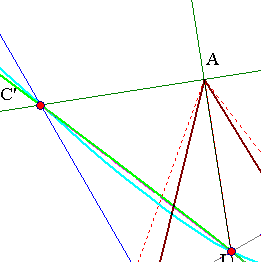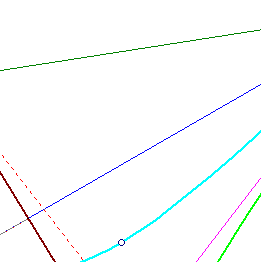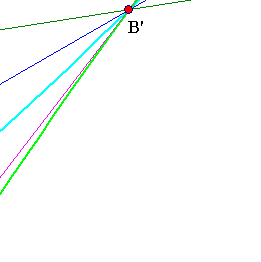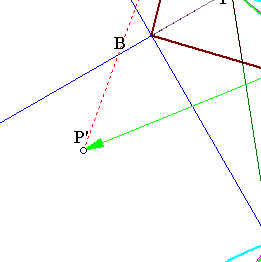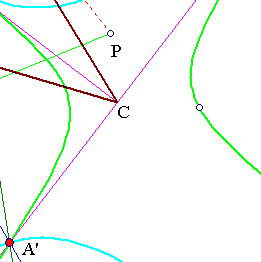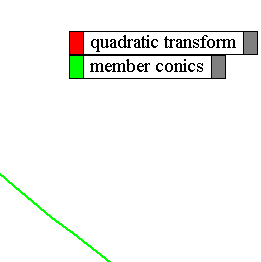The proof, modulo the remarks made above, is trivial. Apply the definition of the quadratic transformation by taking the polars of P with respect to c1, c2. For example, the polar L1 = AP' of P with respect to c1(AA',AB') and line L = AP are harmonic conjugates with respect to AA', AB', which are orthogonal. Hence (see Harmonic_Bundle.html ) the angle of (L, L1) is bisected by lines (AA', AB'). Analogous results are obtained by joining P, P' with the remaining vertices B and C. This completes the proof.
Although the property has a simple proof. The context involves several interesting ideas studied in the references given below.